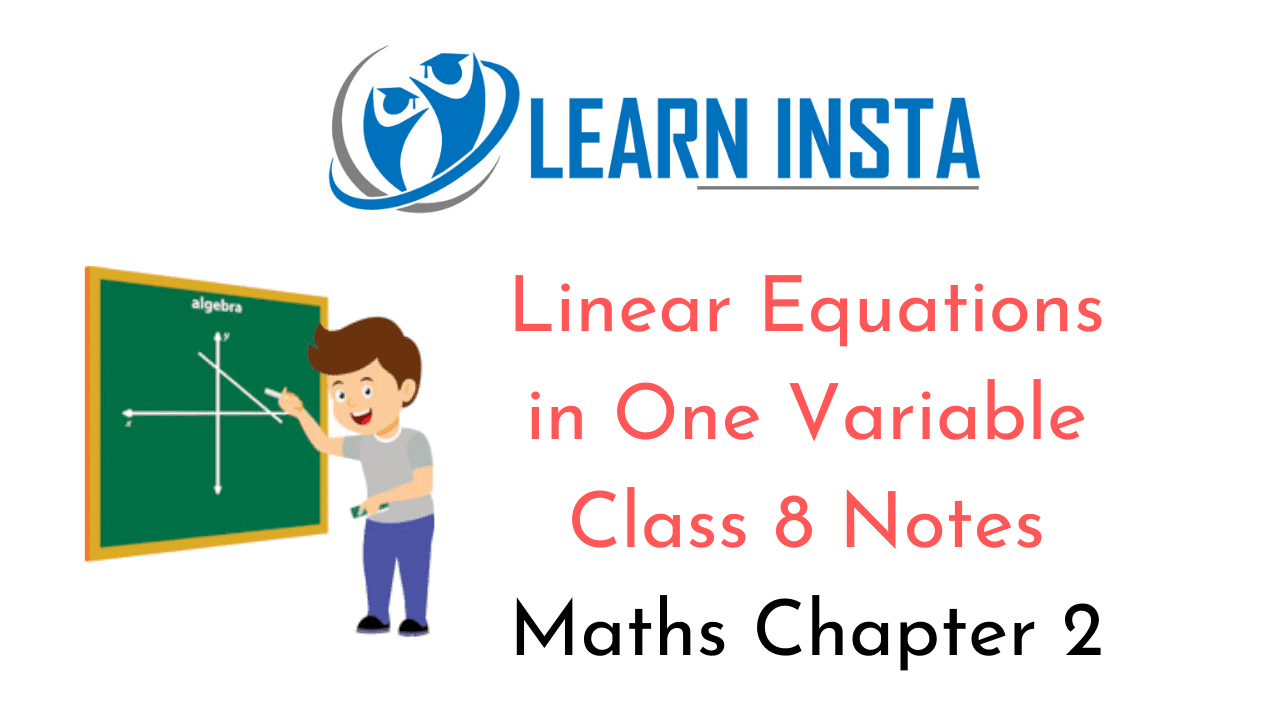On this page, you will find Linear Equations in One Variable Class 8 Notes Maths Chapter 2 Pdf free download. CBSE NCERT Class 8 Maths Notes Chapter 2 Linear Equations in One Variable will seemingly help them to revise the important concepts in less time.

## CBSE Class 8 Maths Chapter 2 Notes Linear Equations in One Variable

### Linear Equations in One Variable Class 8 Notes Conceptual Facts

1. Statement of equality containing one or more variable (unknown quantity) is called an equation.
For example: 3x – 5 = 6, x – 3y = 7, $$\frac{x}{2}+\frac{y}{3}=5$$ , x2 + 5 = y2 are all equations.

2. Linear equations of one variable contains only one variable.
For example: $$3 x-1=\frac{1}{2}, 5-x=\frac{3}{2}, \frac{6 x}{5}-3=0$$

3. The value of the variable which makes an equation true is called the solution of the equation.
x2 + y2 = 7 type equations are not linear equations.
(i) Rules for solving an equation.

• Same number can be added to both sides of equality sign (=).
• Same number can be subtracted from both the sides of equality sign (=).
• Same number can be multiplied to both the sides of equality sign (=).
• Both sides of the equation can be divided by the same number.

For example:
3x – 4 = 7
⇒ 3x- 4 + 4- 7+ 4 (adding 4 to both sides)
⇒ 3x = 11
⇒ 3x÷3 = 11÷3 (dividing the both sides by 3)
$$x=\frac{11}{3}$$
Thus, $$x=\frac{11}{3}$$ is the solution or root of the equation.

(ii) Rule of transpositions: Any term can be transposed from one side of the equation to the other side by changing its sign, i.e. (+) to (-), (-) to (+), (x) to (-r) and (v) to (x).